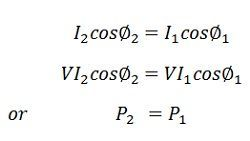# Power Factor Improvement

If the power factor is low or poor, it is necessary to improve or correct it. It may be improved by injecting a leading current into the circuit so as to neutralize the effect of lagging current. The power factor may be improved by using static capacitors or synchronous motors.

### Power factor correction by static capacitors

Consider an inductive load consisting of a resistor R and an inductor L connected to an AC supply. The circuit and phasor diagrams are shown in the figure.

V – supply voltage.
φ1 – phase angle by which the current I1 lags behind the voltage
cosφ1 – original power factor

Let the capacitor C be placed in parallel with the load. It will take a leading current  I from the supply. The circuit and phasor diagrams are shown in the figure.The total I2 drawn from the supply will be equal to the phasor sum of I1 and Ic that is

###Conclusions

• The phase angle of I2 is φ2. It is seen from the phasor diagram that the φ is less than φ1, and hence, cosφ2 is greater than cosφ1. In other words, the power is improved from cosφ1 to cosφ2.
• The new current supply from the supply is less than the load current I1, i.e., I2> I1. The new current is given by the equation• By connecting a capacitor in parallel with an inductive load, the power factor is improved, and the current from the supply is reduced without altering either current or power taken by the load.This relation shows that the power taken from the supply has not altered.

### 4 thoughts on “Power Factor Improvement”

1. Thank you for the knowledge shared.
I need information on how to go about these;

1. How can I connect capacitors to build up a capacitor bank?
2. I need a capacitor bank to carry out experiment on power factor synchronization (EEE 345)?
Thanjs
Cheers

2. Thank you for the knowledge shared.
I need information on how to go about these;

1. How can I connect capacitors to build up a capacitor bank?
2. I need a capacitor bank to carry out experiment on power factor synchronization (EEE 345)?
Thanks
Cheers

3. How and where can I get Capacitor bank sizing and ratings?
Kindly supply this info for me.
Cheers